Divisible

Mathematical Induction
Concept wise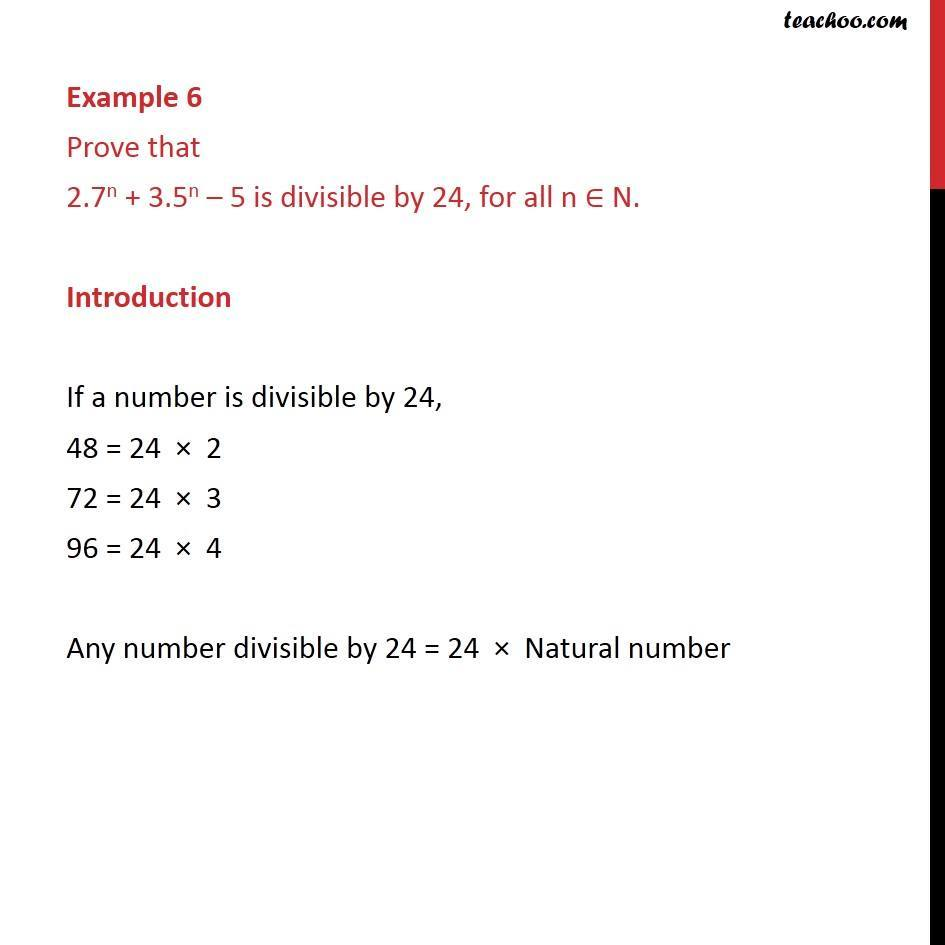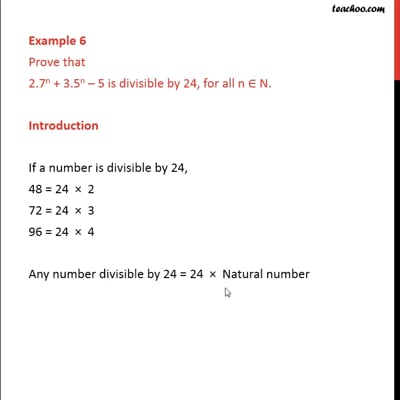This video is only available for Teachoo black users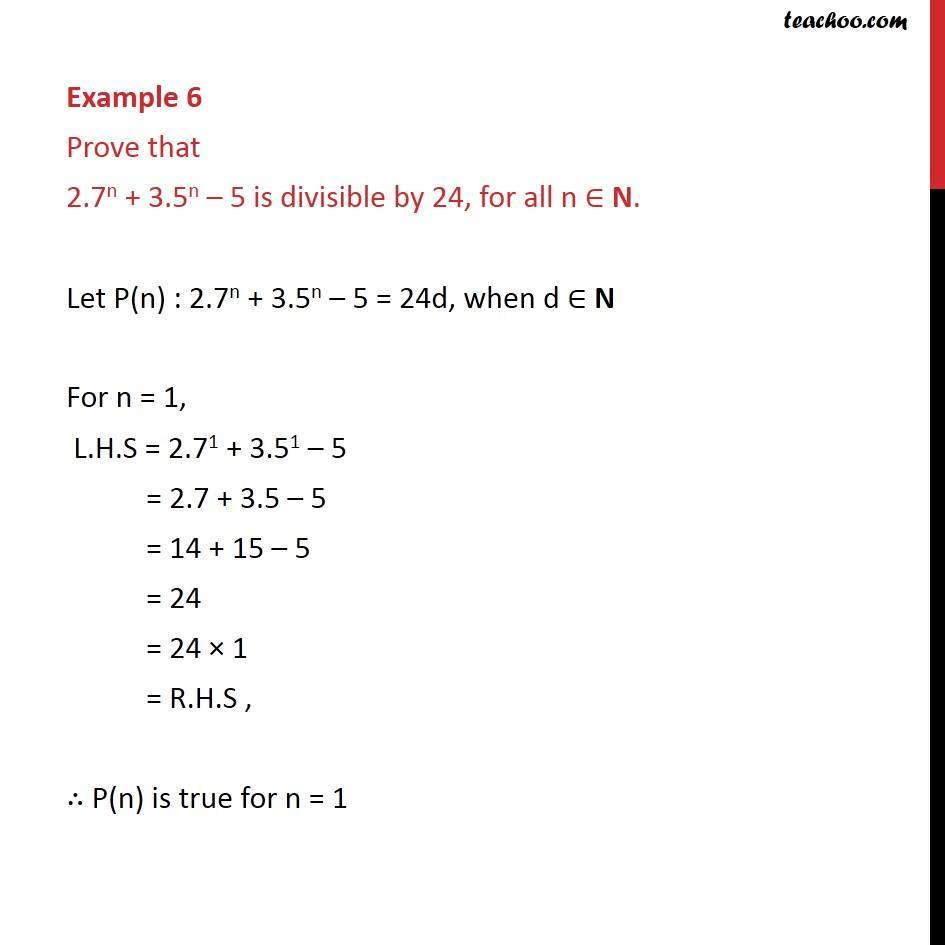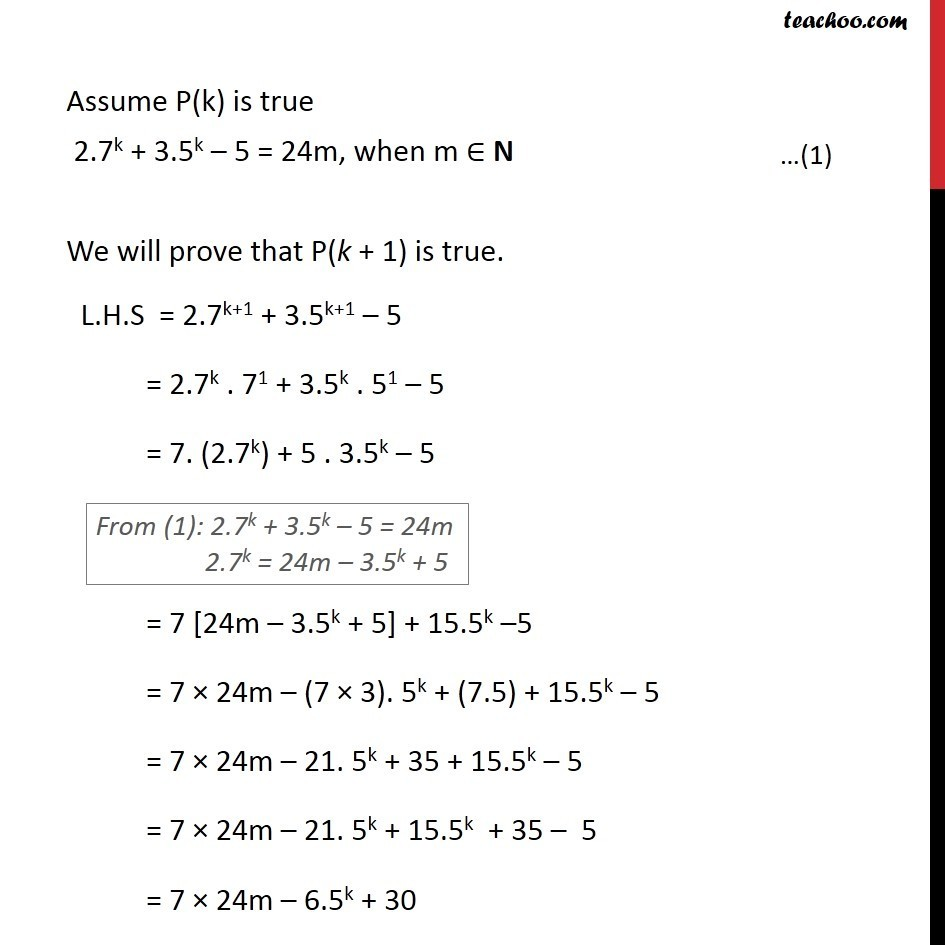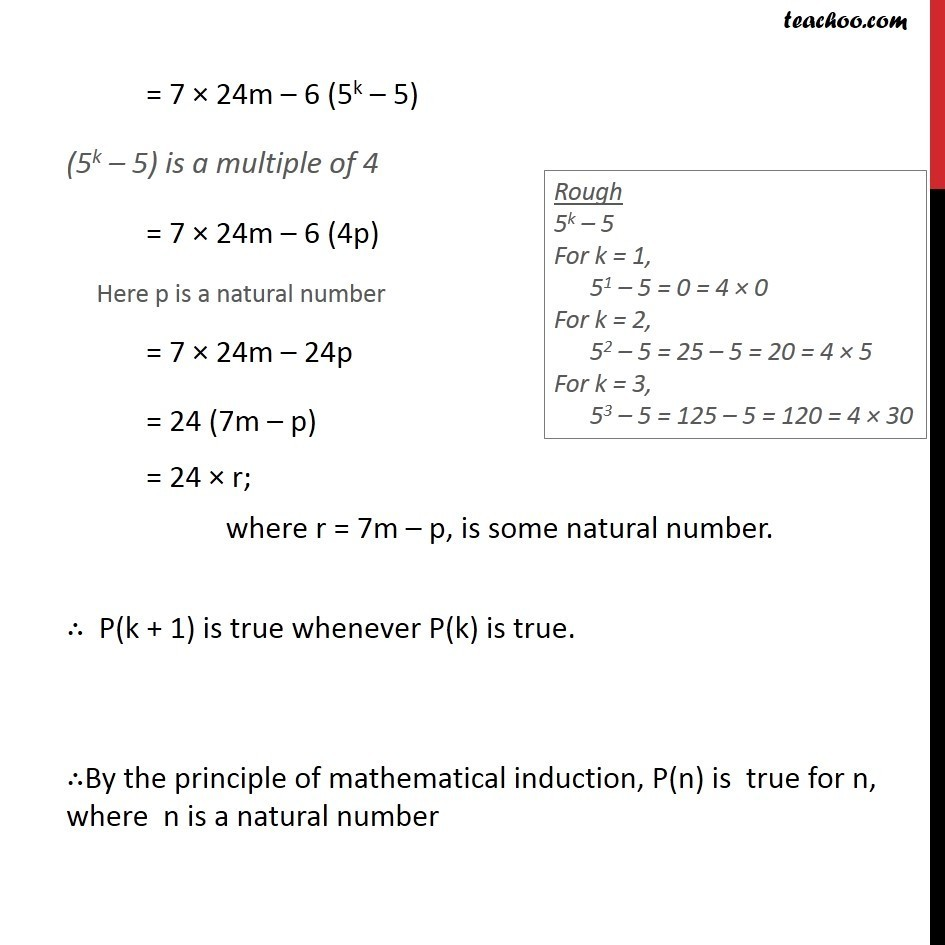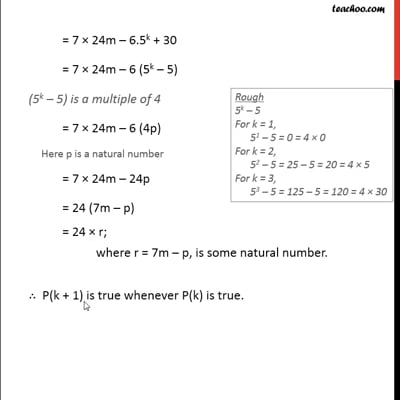This video is only available for Teachoo black users

Learn in your speed, with individual attention - Teachoo Maths 1-on-1 Class

### Transcript

Example 6 Prove that 2.7n + 3.5n 5 is divisible by 24, for all n N. Introduction If a number is divisible by 24, 48 = 24 2 72 = 24 3 96 = 24 4 Any number divisible by 24 = 24 Natural number Example 6 Prove that 2.7n + 3.5n 5 is divisible by 24, for all n N. Let P(n) : 2.7n + 3.5n 5 = 24d, when d N For n = 1, L.H.S = 2.71 + 3.51 5 = 2.7 + 3.5 5 = 14 + 15 5 = 24 = 24 1 = R.H.S , P(n) is true for n = 1 Assume P(k) is true 2.7k + 3.5k 5 = 24m, when m N We will prove that P(k + 1) is true. L.H.S = 2.7k+1 + 3.5k+1 5 = 2.7k . 71 + 3.5k . 51 5 = 7. (2.7k) + 5 . 3.5k 5 = 7 [24m 3.5k + 5] + 15.5k 5 = 7 24m (7 3). 5k + (7.5) + 15.5k 5 = 7 24m 21. 5k + 35 + 15.5k 5 = 7 24m 21. 5k + 15.5k + 35 5 = 7 24m 6.5k + 30 = 7 24m 6 (5k 5) (5k 5) is a multiple of 4 = 7 24m 6 (4p) = 7 24m 24p = 24 (7m p) = 24 r; where r = 7m p, is some natural number. P(k + 1) is true whenever P(k) is true. By the principle of mathematical induction, P(n) is true for n, where n is a natural number